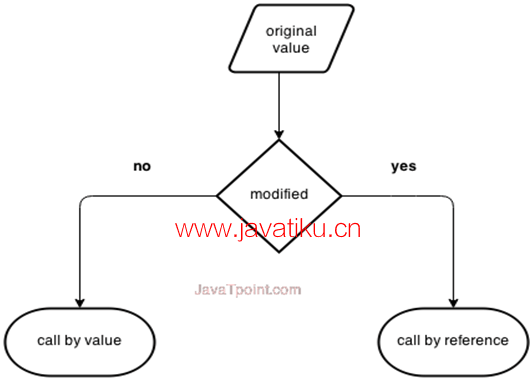# C++教程-C++按值传递和按引用传递## 按值传递和按引用传递### 按值传递

``````cppCopy code
#include <iostream>
using namespace std;
void change(int data);
int main()
{
int data = 3;
change(data);
cout << "Value of the data is: " << data << endl;
return 0;
}
void change(int data)
{
data = 5;
}``````

``Value of the data is: 3``

### 按引用传递

``````cppCopy code
#include<iostream>
using namespace std;
void swap(int *x, int *y)
{
int temp;
temp = *x;
*x = *y;
*y = temp;
}
int main()
{
int x = 500, y = 100;
swap(&x, &y);  // 将值传递给函数
cout << "Value of x is: " << x << endl;
cout << "Value of y is: " << y << endl;
return 0;
}``````

``````Value of x is: 100
Value of y is: 500``````

### 按值传递和按引用传递在C++中的区别：

1传递给函数的是值的副本传递给函数的是值的地址
2函数内部的修改不会反映在其他函数中函数内部的修改会反映在函数的外部
3实际参数和形式参数在不同的内存位置中创建实际参数和形式参数在相同的内存位置中创建